This site uses cookies. By continuing to browse the ConceptDraw site you are agreeing to our Use of Site Cookies.
ConceptDraw Samples | Business Charts — Bar and Histogram

# Bar Charts

Samples of Business Diagrams (Bar Graphs, Column Charts and Histograms) are created with ConceptDraw DIAGRAM diagramming and vector drawing software enhanced with business diagramming solutions from ConceptDraw Solution Park. The ConceptDraw DIAGRAM offers you a variety of diagramming tools, and solutions from the Solution Park provide diagram templates and libraries of ready-made charts and shapes for quick and simple drawing professional-quality business diagrams. ConceptDraw DIAGRAM provides export of vector graphic multipage documents into multiple file formats: vector graphics (SVG, EMF, EPS), bitmap graphics (PNG, JPEG, GIF, BMP, TIFF), web documents (HTML, PDF), PowerPoint presentations (PPT), Adobe Flash (SWF).

## Tutorials and Solutions:

Video Tutorials: ConceptDraw Solution Park

Solutions: Basic Diagramming for ConceptDraw DIAGRAM

## Sample 1: Double Bar Graph — Occupational Profile of Volunteers and Paid Workforce

Double Bar Graph sample: Occupational profile of volunteers and paid workforce.

This example is created using ConceptDraw DIAGRAM diagramming software enhanced with
solution Basic Diagramming from ConceptDraw Solution Park.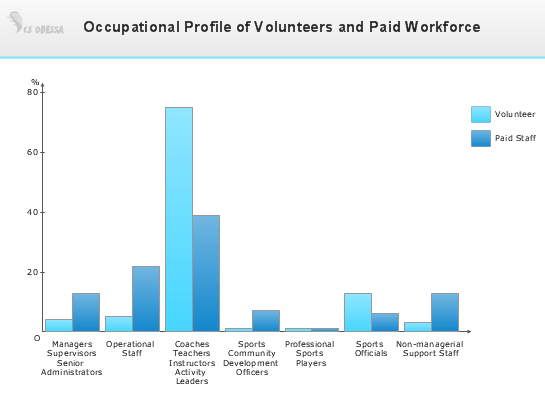## Sample 2: Double Bar Graph — Urban Population Growth and Projections

Double Bar Graph sample: Urban population growth and projections.

This example is created using ConceptDraw DIAGRAM diagramming software enhanced with
solution Basic Diagramming from ConceptDraw Solution Park.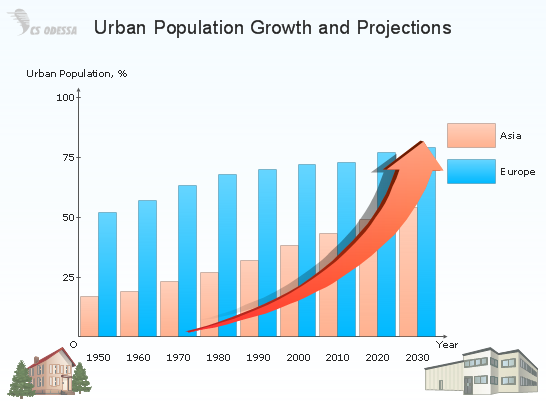## Sample 3: Percent Bar Chart — Age Composition of the Population

Percent Bar Chart sample: Age composition of the population.

This example is created using ConceptDraw DIAGRAM diagramming software enhanced with
solution Basic Diagramming from ConceptDraw Solution Park.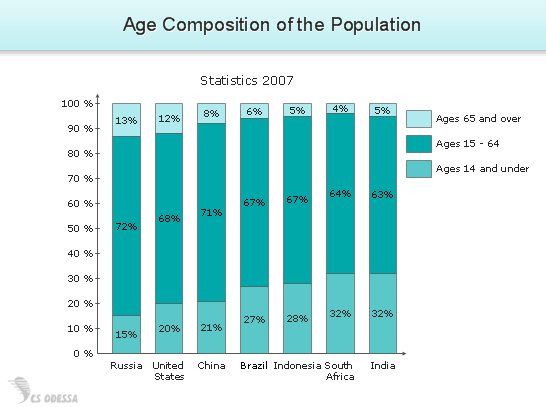## Sample 4: Percentage Bar Chart — Daily Sales Percentage

Normalized Stacked Bar Chart sample: Daily sales percentage.

This example is created using ConceptDraw DIAGRAM diagramming software enhanced with
solution Basic Diagramming from ConceptDraw Solution Park.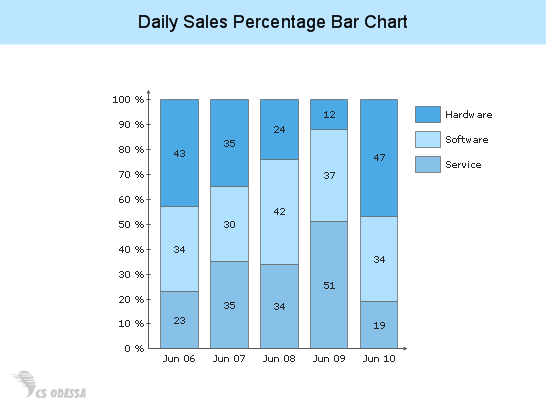## Sample 5: Percent Bar Chart — Different Approaches to the Camera by Gender

Normalized Stacked Bar Chart sample: Different approaches to the camera by gender.

This example is created using ConceptDraw DIAGRAM diagramming software enhanced with
solution Basic Diagramming from ConceptDraw Solution Park.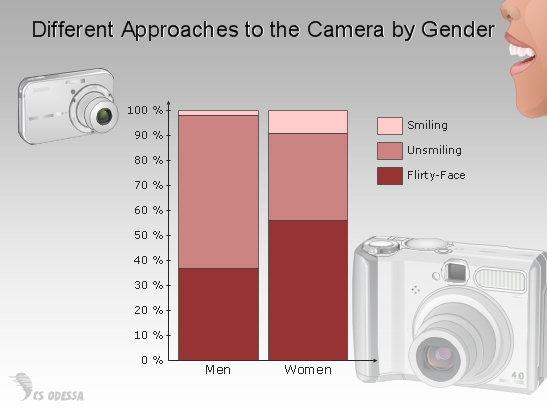## Sample 6: Percent Stacked Bar Chart — Market Share Percentage

Normalized Stacked Bar Chart sample: Market share percentage.

This example is created using ConceptDraw DIAGRAM diagramming software enhanced with
solution Basic Diagramming from ConceptDraw Solution Park.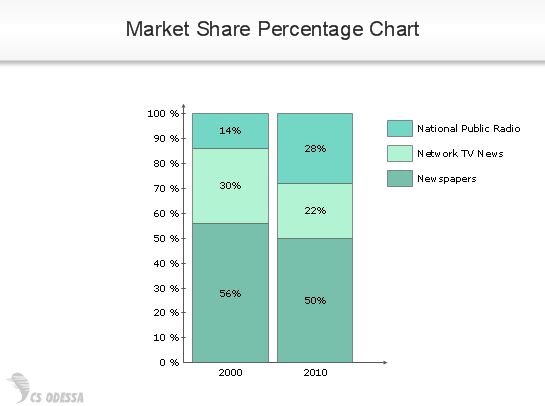## Sample 7: Bar Graph Horizontal

Horizontal Bar Chart sample: The Most Spoken Languages of the World.

This example is created using ConceptDraw DIAGRAM diagramming software enhanced with
solution Basic Diagramming from ConceptDraw Solution Park.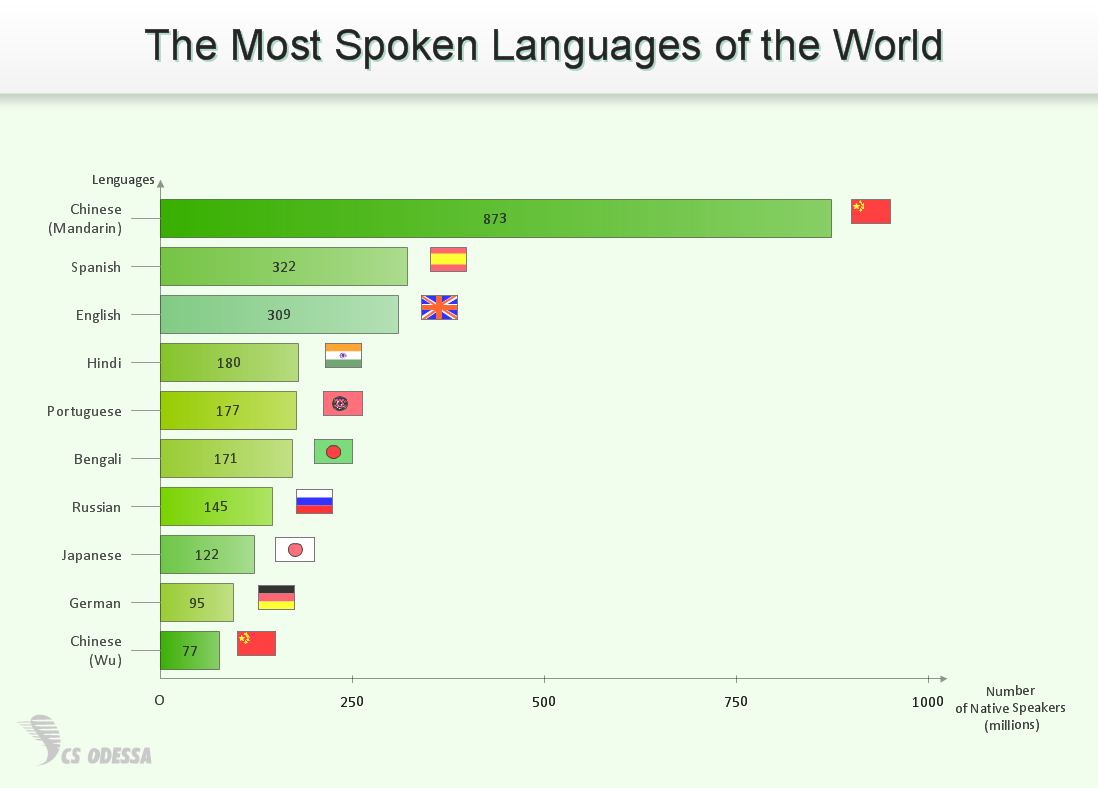## Sample 8: Vertical Bar Graph Sample

Column Chart sample: Average Monthly Rainfall.

This example is created using ConceptDraw DIAGRAM diagramming software enhanced with
solution Basic Diagramming from ConceptDraw Solution Park.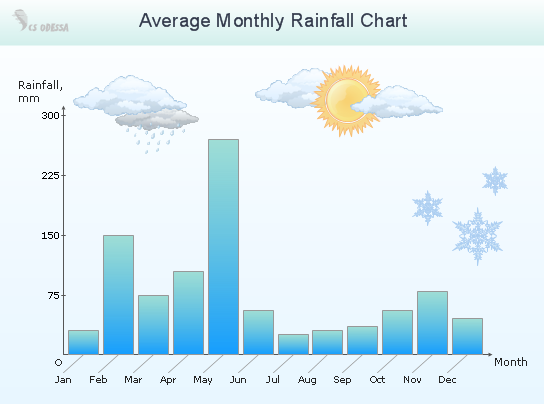## Sample 9: Vertical Bar Graph

Column Chart sample: Colleagues' Birthdays by Month.

This example is created using ConceptDraw DIAGRAM diagramming software enhanced with
solution Basic Diagramming from ConceptDraw Solution Park.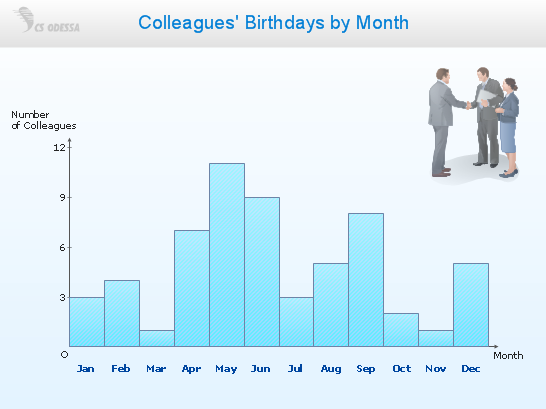## Sample 10: Stacked Bar Chart

Stacked Bar Chart example (3 series): Sales.

This example is created using ConceptDraw DIAGRAM diagramming software enhanced with
solution Basic Diagramming from ConceptDraw Solution Park.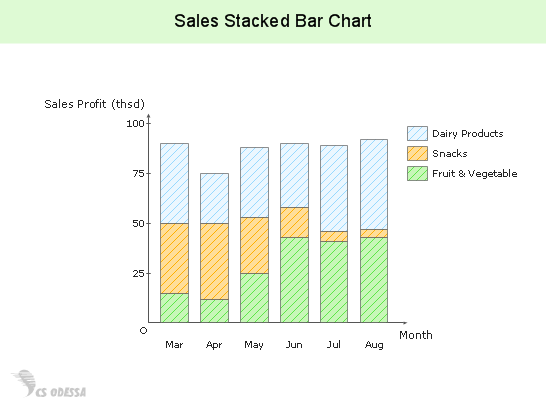## Sample 11: Histogram — Employee Salary

Histogram sample: Employee salary.

This example is created using ConceptDraw DIAGRAM diagramming software enhanced with
solution Basic Diagramming from ConceptDraw Solution Park.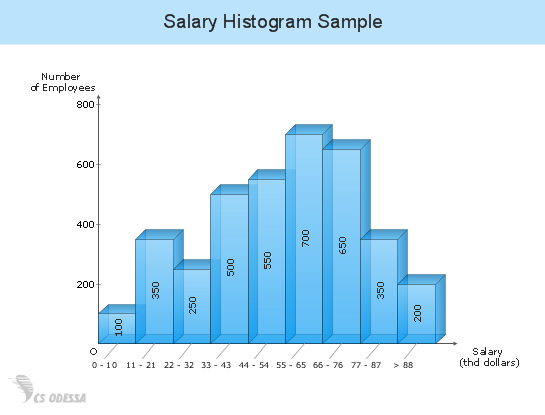## Sample 12: Divided Bar Graph — The Number of Jobs in Design Careers

Divided Bar Graph sample: The number of jobs in design careers.

This example is created using ConceptDraw DIAGRAM diagramming software enhanced with
solution Basic Diagramming from ConceptDraw Solution Park.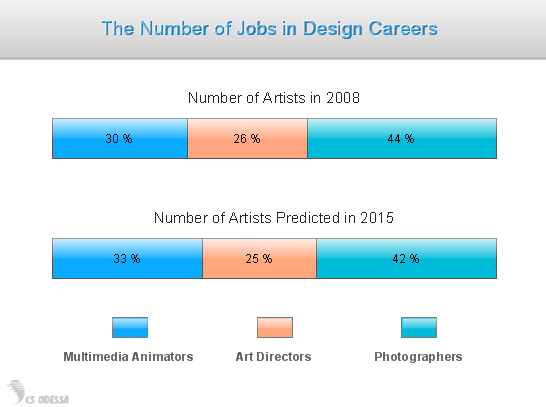## Sample 13: Divided Bar Charts — A Survey of Students' Preferences

Divided Bar Charts sample: A survey of students' preferences.

This example is created using ConceptDraw DIAGRAM diagramming software enhanced with
solution Basic Diagramming from ConceptDraw Solution Park.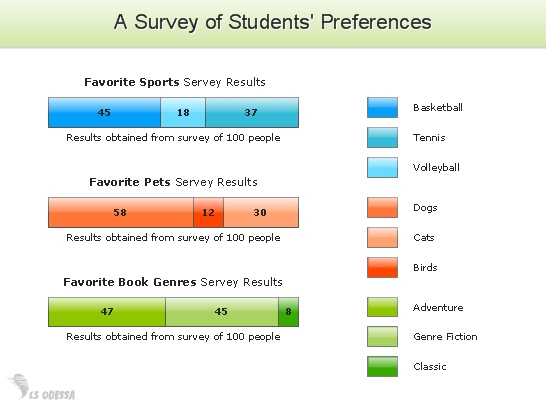All samples are copyrighted CS Odessa's.

Usage of them is covered by Creative Commons “Attribution Non-Commercial No Derivatives” License.

The text you can find at: https://creativecommons.org/licenses/by-nc-nd/3.0